Question

# In Einstein's photoelectric experiment, the kinetic energy (K.E.) of an electron displaced from a metal by...

In Einstein's photoelectric experiment, the kinetic energy (K.E.) of an electron displaced from a metal by a photon was the difference between the energy of a photon and a threshold energy value for the metal. If you were to perform a similar experiment with lead, which has a threshold energy of 6.81 × 10⁻¹⁹ J, and ultraviolet light of wavelength 38.5 nm, what would be the speed (v) of the dislodged electron? (K.E. = 1/2mv². The mass of the electron = 9.109 × 10⁻³¹ kg.)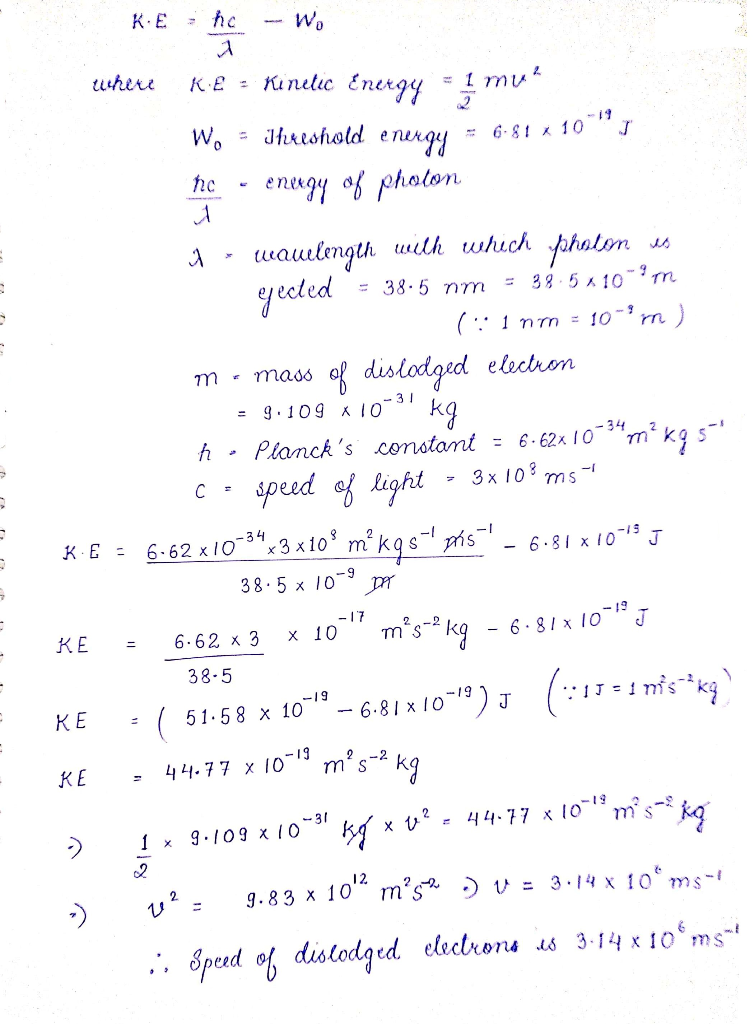Speed of dislodged electron is 3.14×10^6 m/s

Dear student,

#### Earn Coins

Coins can be redeemed for fabulous gifts.

Similar Homework Help Questions
• ### In a photoelectric effect experiment, a photon with energy of 1.25x10-18J is absorbed, causing ejection of...

In a photoelectric effect experiment, a photon with energy of 1.25x10-18J is absorbed, causing ejection of an electron with kinetic energy of 2.55x10-19J. a. Determine the wavelength (in nm) associated with the absorbed particle. b. Determine the wavelength (in nm) associated with the emitted particle.

• ### A metal surface has a minimum binding energy of 2.34x10-19 J/electron. An incident light of 345...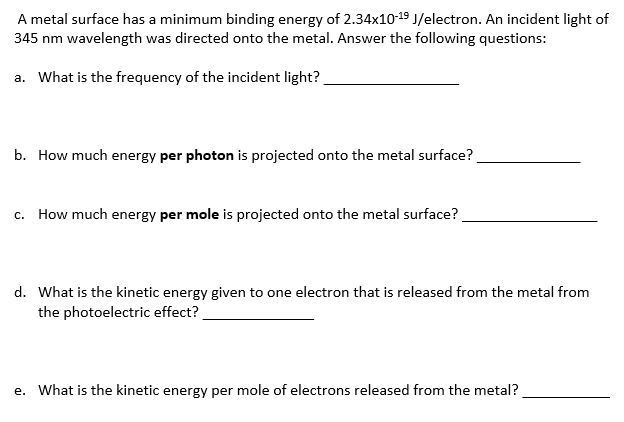A metal surface has a minimum binding energy of 2.34x10-19 J/electron. An incident light of 345 nm wavelength was directed onto the metal. Answer the following questions: a. What is the frequency of the incident light? b. How much energy per photon is projected onto the metal surface? c. How much energy per mole is projected onto the metal surface? d. What is the kinetic energy given to one electron that is released from the metal from the photoelectric effect?...

• ### 1.(16 pts) (1) In a photoelectric experiment, Molybdenum must absorb radiation having a frequency of 1.09...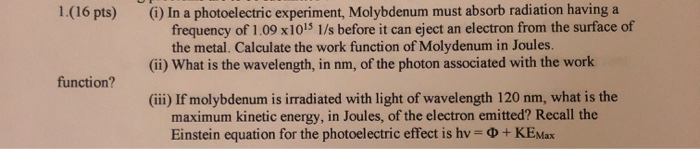1.(16 pts) (1) In a photoelectric experiment, Molybdenum must absorb radiation having a frequency of 1.09 x 1015 1/s before it can eject an electron from the surface of the metal. Calculate the work function of Molydenum in Joules. (ii) What is the wavelength, in nm, of the photon associated with the work function? (iii) If molybdenum is irradiated with light of wavelength 120 nm, what is the maximum kinetic energy, in Joules, of the electron emitted? Recall the Einstein...

• ### 1.(16 pts) (i) In a photoelectric experiment, Molybdenum must absorb radiation having a frequency of 1.09...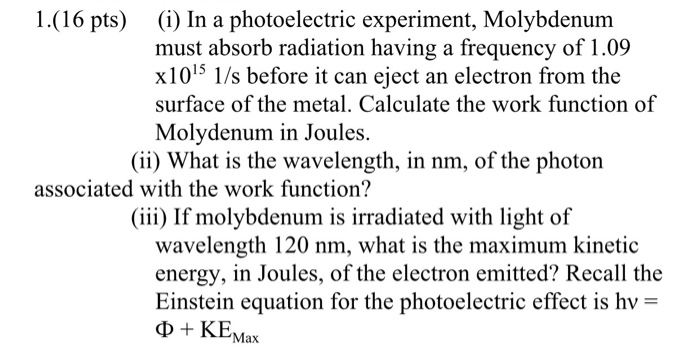1.(16 pts) (i) In a photoelectric experiment, Molybdenum must absorb radiation having a frequency of 1.09 x105 1/s before it can eject an electron from the surface of the metal. Calculate the work function of Molydenum in Joules (ii) What is the wavelength, in nm, of the photon associated with the work function? (iii) If molybdenum is irradiated with light of wavelength 120 nm, what is the maximum kinetic energy, in Joules, of the electron emitted? Recall the Einstein equation...

• ### The photoelectric work function energy for gold metal is 4.82 eV (per electron). What wavelength of...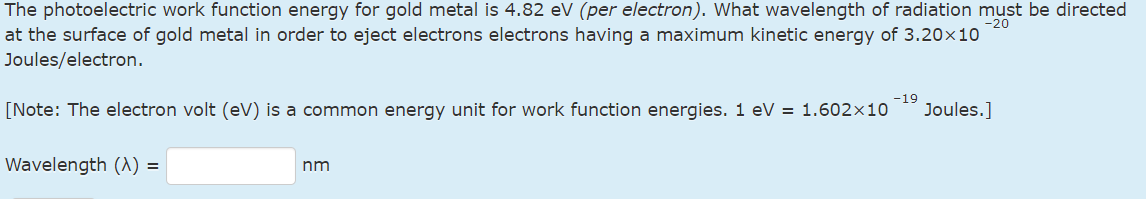The photoelectric work function energy for gold metal is 4.82 eV (per electron). What wavelength of radiation must be directed at the surface of gold metal in order to eject electrons electrons having a maximum kinetic energy of 3.20×10 –20 Joules/electron. [Note: The electron volt (eV) is a common energy unit for work function energies. 1 eV = 1.602×10 –19 Joules.] -20 The photoelectric work function energy for gold metal is 4.82 eV (per electron). What wavelength of radiation must...

• ### What is the maximum kinetic energy of an ejected electron if platinum metal is irradiated with...

What is the maximum kinetic energy of an ejected electron if platinum metal is irradiated with 166-nm light? The threshold wavelength for a platinum metal surface is 212 nm. Give your answer accurate to three significant figures. Use exponential notation (e.g. 1.23e-19).

• ### The wavelength required to dislodge electrons from a certain metal via the photoelectric effect is 350 nm. When light of an unknown wavelength is shined upon the metal, electrons with kinetic ene...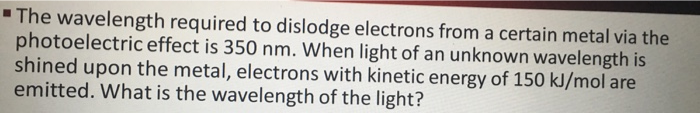The wavelength required to dislodge electrons from a certain metal via the photoelectric effect is 350 nm. When light of an unknown wavelength is shined upon the metal, electrons with kinetic energy of 150 kJ/mol are emitted. What is the wavelength of the light? The wavelength required to dislodge electrons from a certain metal via the photoelectric effect is 350 nm. When light of an unknown wavelength is shined upon the metal, electrons with kinetic energy of 150 kJ/mol are...

• ### The photoelectric work function energy for manganese metal is 3.76 eV (per electron). What wavelength of...

The photoelectric work function energy for manganese metal is 3.76 eV (per electron). What wavelength of radiation must be directed at the surface of manganese metal in order to eject electrons electrons having a maximum kinetic energy of 2.00×10 –19 Joules/electron. [Note: The electron volt (eV) is a common energy unit for work function energies. 1 eV = 1.602×10 –19 Joules.] Wavelength (λ) = Answer nm

• ### Thanks! 5. (10 pts) (a) Einstein's explanation of the photoelectric effect launched the study of quantum...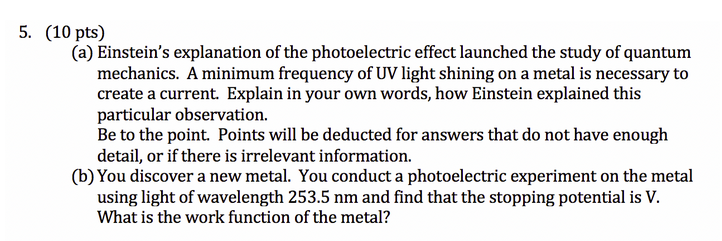Thanks! 5. (10 pts) (a) Einstein's explanation of the photoelectric effect launched the study of quantum mechanics. A minimum frequency of UV light shining on a metal is necessary to create a current. Explain in your own words, how Einstein explained this particular observation. Be to the point. Points will be deducted for answers that do not have enough detail, or if there is irrelevant information. (b) You discover a new metal. You conduct a photoelectric experiment on the metal...

• ### 10) A photoelectric effect experiment is done using light of some wavelength 2. The photoelectrons are...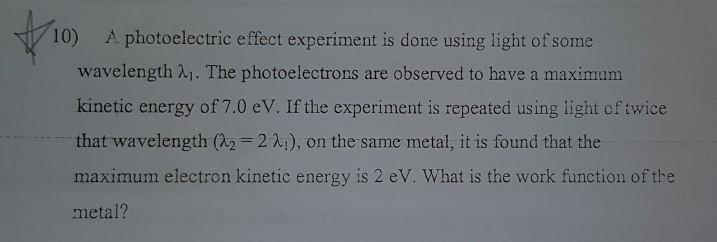10) A photoelectric effect experiment is done using light of some wavelength 2. The photoelectrons are observed to have a maximum kinetic energy of 7.0 eV. If the experiment is repeated using light of twice that wavelength (22 =221), on the same metal, it is found that the maximum electron kinetic energy is 2 eV. What is the work function of the metal?